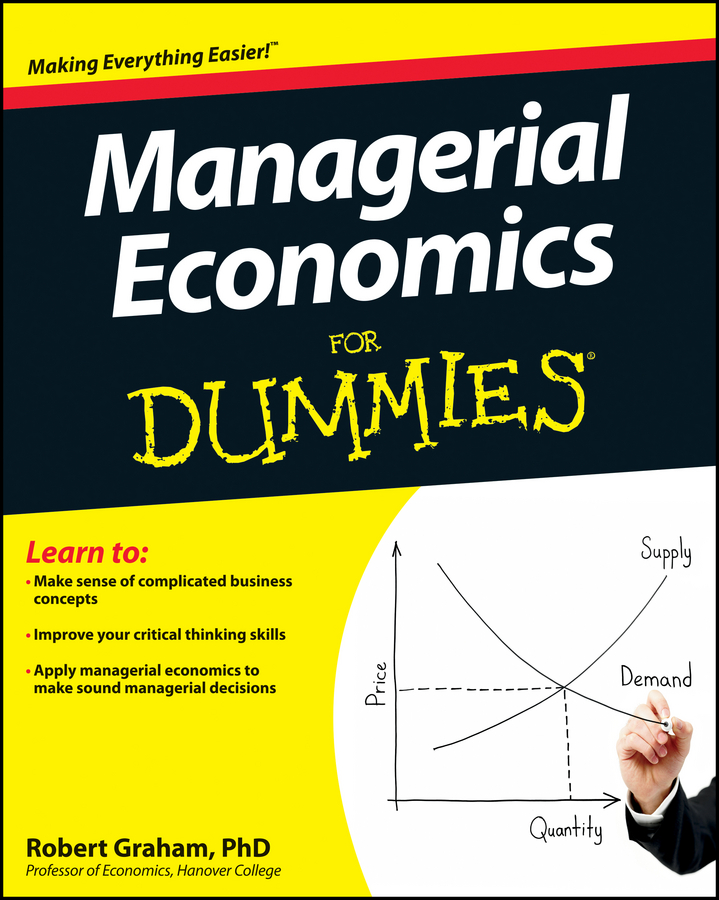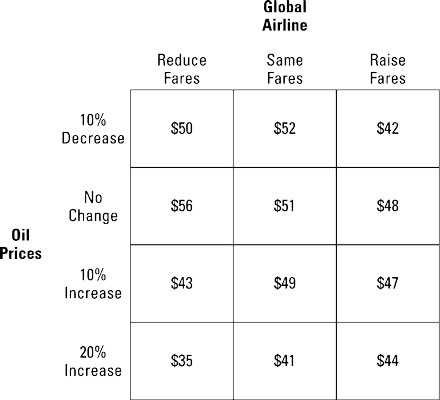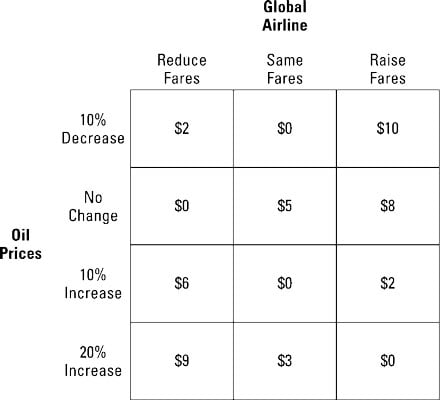##### Managerial Economics For DummiesThe mini-max regret criterion in managerial economics bases business decisions on the maximum regret associated with each action. Regret measures the difference between each action’s payoff for a given state of nature and the best possible payoff for that state of nature.

You choose the action that has the smallest maximum regret.Given the information above, Global Airlines uses the mini-max regret criterion to determine what fare to set given it doesn’t know what’s going to happen to the price of oil. To apply the mini-max regret criterion, Global takes the following steps:

1. Determine the regret for a 10-percent decrease in the price of oil.

For a 10-percent decrease in the price of oil, the best payoff is \$52 million with same fares. The regret for reducing fares is \$2 million, \$52 – \$50, and the regret for raising fares is \$10 million, \$52 – \$42. The maximum regret is \$10 million.

2. Determine the regret for no change in the price of oil.

For no change in the price of oil, the best payoff is \$56 million with reduced fares. The regret for keeping the same fares is \$5 million, \$56 – \$51, and the regret for raising fares is \$8 million, \$56 – \$48. The maximum regret is \$8 million.

3. Determine the regret for a 10-percent increase in the price of oil.

For a 10-percent increase in the price of oil, the best payoff is \$49 million with the same fares. The regret for reducing fares is \$6 million, \$49 – \$43, and the regret for raising fares is \$2 million, \$49 – \$47. The maximum regret is \$6 million.

4. Determine the regret for a 20-percent increase in the price of oil.

For a 20-percent increase in the price of oil, the best payoff is \$44 million with raising fares. The regret for reducing fares is \$9 million, \$44 – \$35, and the regret for keeping the same fares is \$3 million, \$44 – \$41. The maximum regret is \$9 million.

5. Choose the action with the minimum or smallest maximum regret.

The illustration summarizes the regrets for each action. The maximum regret associated with reducing fares is \$9 million. The maximum regret in the same-fares column is \$5 million, and the maximum regret you see with raising fares is \$10 million. Global Airlines should charge the same fares because its maximum regret of \$5 million is smaller than the maximum regret associated with any other action.

The primary advantage of the mini-max regret decision criterion is its relative simplicity. This criterion’s disadvantages include its emphasis on the worst possible outcome, and it ignores risk as represented by an outcome’s probability of occurring.